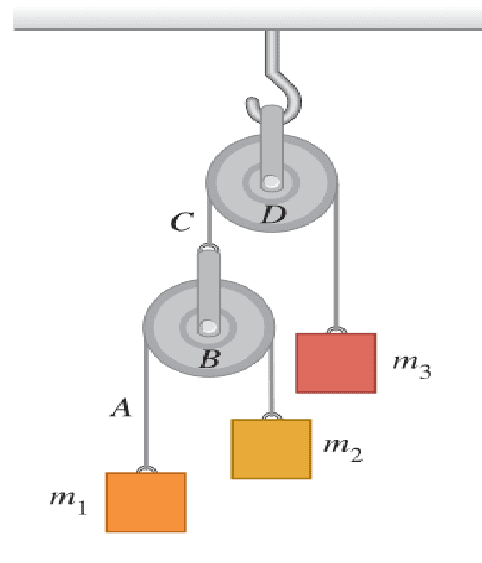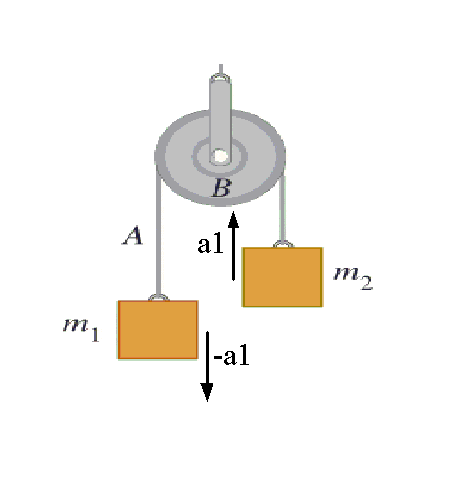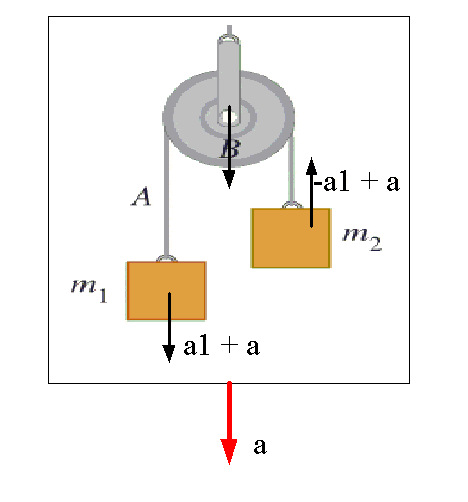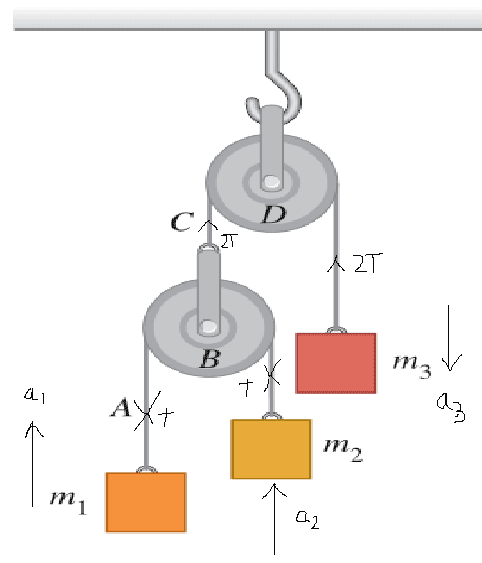# Acceleration of massless pulley and blocks

• Bruneleski
The average of the two accelerations must be the same as the acceleration of the pulley B system as a whole, namely, the average of the two accelerations is the acceleration of the pulley B system, namely, a.f

## Homework Statement

Consider a situationI'm curious why is that the massless pulley's acceleration is just the avarage of acceleration of m1 and that of m2?
ab=(a1+a2)/2

## Homework Equations

Forces acting on pulley are Tc-2Ta=0 (because pulley is massless, force can't give it acceleration)
Therefore tensions are Tc=2Ta
But, Ta-m1g=m1a1
and Ta-m2g=m2a2
[/B]

## The Attempt at a Solution

Intuitively it makes sense.But I can't find the equation that describes this relationship.[/B]

Consider the position of B as function of the positions of the masses and the string lengths. There is no need to consider forces, this is a simple mechanical constraint.

Does it have something to do with notion that string on the left side is twice the length that on the right?

The absolute lengths of the strings is not relevant, they just have to stay constant.

What I meant was that m1 is placed lower than m2.Anyway, I still don't get why ab=(a1+a2)/2 holds.Can you please write those equations?

Let the string lengths be L1, L2, L=L1+L2. Differentiate twice.

@haruspex I don't know what you mean by that.What are L1 and L2 respectively? Why differentiate?

@haruspex I don't know what you mean by that.What are L1 and L2 respectively?
The lengths either side of the pulley, i.e. from pulley to m1 and pulley to m2.
Why differentiate?
Because you are interested in accelerations.

L''=L1''+L2'', this gives a=a1+a2 .I'm not sure how that helps. But based on initial picture, L1 =2L 2 ,but how this relates to acceleration?
And why is L related to acceleration of a pulley?

L''=L1''+L2'', this gives a=a1+a2 .I'm not sure how that helps. But based on initial picture, L1 =2L 2 ,but how this relates to acceleration?
And why is L related to acceleration of a pulley?
L is the total length of the string, so what is L"?
L1" and L2" represent relative accelerations of objects in the diagram. Can you figure out what they are?

L1'' is acceleration of m1 and L2'' of m2.L'' is then aceleration of pulley,right?But how that gives desired relationship?

L1'' is acceleration of m1 and L2'' of m2. L'' is then acceleration of pulley, right?
No. As I wrote, L1" and L2" are relative accelerations.
Think of L1 as a relative height, i.e. the difference in height of two objects. Which two objects?
As for L", does L vary?

L is constant (string). So L'' is zero.
But L1 and L2 do vary because of m1 is not equal m2.
Then pulley goes distance L1 relative to m1, hence L1'' is acceleration of pulley relative to m1.Likewise, L2'' is acceleration of pulley relative to m2.Right?

L is constant (string). So L'' is zero.
But L1 and L2 do vary because of m1 is not equal m2.
Then pulley goes distance L1 relative to m1, hence L1'' is acceleration of pulley relative to m1.Likewise, L2'' is acceleration of pulley relative to m2.Right?
Yes. Can you now get the result you are after?

Unfortunately, I can't figure out how to put this to use.Considering I found out relations between acc. of pulley and acc. both m1 and m2 I should be able to relate those 3.
So I just need equation that includes accelerations of those 3 objects. This however I know ab=(a1+a2)/2.
But how do I get a1 and a2 from this stuff above?I understand conceptually but can't write it down.

This however I know ab=(a1+a2)/2.
I'm confused... I thought that was what you were trying to prove.

Yes,indeed i was trying to prove that.But I don't know how a1 and a2 come up from what we discussed above.(or whole equation for that matter)

Yes,indeed i was trying to prove that.But I don't know how a1 and a2 come up from what we discussed above.(or whole equation for that matter)
Taking all accelerations as positive upwards (say), write ab in terms of a1 and L1". Do the same for mass 2. Eliminate L1" and L2" using L''=L1''+L2".

•Bruneleski
This might be a case where a couple of pictures can help to clarify the situation.

Suppose we isolate the pulley B and the masses m1 and m2 as a system and have an observer ride along with it. The observer decides to count downward acceleration as positive and sees mass m1 accelerating downward with some acceleration +a1 with respect to the pulley, and mass m2 accelerating with acceleration -a1. The accelerations must have the same magnitude because the string has a constant length; however much or fast m1 moves, m2 must move by the same amount and at the same rate as m1 with respect to the pulley.Now another observer in the fixed lab frame of reference looks at the situation (figure below) and notes that the pulley B system as a whole is accelerating downward with some acceleration a. He thus sees m1 accelerating downward with acceleration a + a1, and mass m2 upward with acceleration -a1 + a. What's the average of those two accelerations?•Bruneleski
I have a trick through which you can easily find these types of constraint equations.
For any closed system ##\sum { \vec { T } .\vec { a } }=0## holds. (T.a is the dot product of tension and acceleration )
But there are some condition in which it does not holds.So
##-2Ta_{3}+Ta_{1}+Ta_{2}=0##

So ##a_{3}=\frac{a_{1}+a_{2}}{2}##

As acceleration of ##m_{3}## is ##a_{3}## so acceleration of pulley should also be ##a_{3}##.

There are some limitations regarding the application of this concept. We can use this only when there is no angular motion between string and pulleys.

It's really straightforward kinematics. Let α represent the downward acceleration of mass 1 relative to pulley B and let β represent the downward acceleration of pulley B. The the absolute downward acceleration of mass 1 is then equal to β+α, and the absolute downward acceleration of mass 2 is equal to β-α. What is the average of the absolute accelerations of masses 1 and 2 in terms of α and β?

Chet

•Sho Kano and Bruneleski
@gneill , @Chestermiller Why do you take the avarage acceleration?
I do not "take" anything. You asked us how the average acceleration of the masses is related to the acceleration of the massless pulley B. This is determined by geometry, not by physics. The expressions I wrote apply irrespective of the physics of the problem. Read what I wrote carefully. From geometry, the average acceleration of the two masses has to equal the acceleration of the pulley B. I don't need to write or solve force balance equations to determine this.

Chet

I was deluded by that the whole time.Now it seems obvious
a1=β+α from this β=a1
a2=β-α from this α=β-a2
Then β=a1-β+a2 which gives 2β=a1+a2
and because β=a3 we have a3=(a1+a2)/2
Thanks

•Sho Kano
I was deluded by that the whole time.Now it seems obvious
a1=β+α from this β=a1
a2=β-α from this α=β-a2
Then β=a1-β+a2 which gives 2β=a1+a2
and because β=a3 we have a3=(a1+a2)/2
Thanks
I'm glad it's obvious now, but I hope you saw from my post #18 how to prove it.

•Sho Kano
I'm glad it's obvious now, but I hope you saw from my post #18 how to prove it.
Yes,by differentiating I got the same result.Thanks.Those pics helped a lot though.